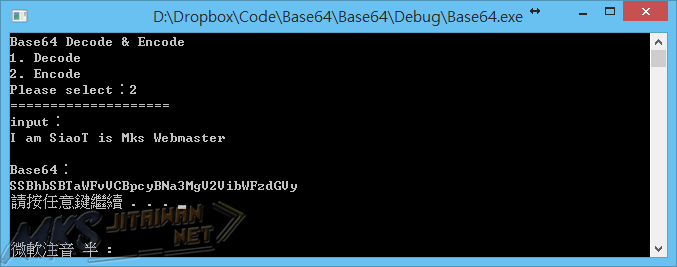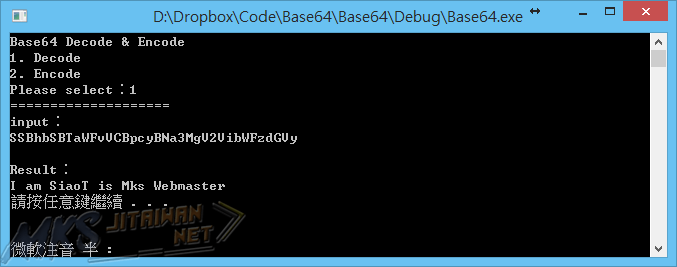# [C/C++] Base64 編解碼程式Base64  編碼語解碼，其實是站長指派的「物件導性程式語言」的小組作業，至於為什麼要出編解碼，是因為站長很喜歡編碼與解碼的題目，算是喜歡玩含有邏輯概念的題目吧，聽說隔壁班有出到 RSA 加密，站長就沒研究了，藉口是沒時間，實際上是懶得再去碰程式了，然後由於久久沒發文，今天拿之前寫的 Base64 編解碼程式來衝一下文章數。

## CODE

```#include <iostream>
#define CODE "ABCDEFGHIJKLMNOPQRSTUVWXYZabcdefghijklmnopqrstuvwxyz0123456789+/"
#define EZ    63
using namespace std;

char E = NULL;
char D;
int out;
void Decode(char i, char x){
if (x == 1){
D = (i << 2);
}else if (x == 2){
cout << char(D | (i >> 4));
D = (i << 4);
}else if (x == 3){
cout << char(D | (i >> 2));
D = (i << 6);
}else if (x == 4){
cout << char(D | i);
}
}

char Encode(char i,char x){
if (x == 1){
out = (i >> 2) | E;
E = (i << 4) & EZ;
return out ;
}else if(x == 2){
out = (i >> 4) | E;
E = (i << 2) & EZ;
return out;
}else if (x == 3){
out = (i >> 6) | E;
E = i & EZ;
return out;
}
}

int main(){
int i, x = 1;
int key;
char* in = new char;
cout << "Base64 Decode & Encode" << endl;
cout << "1. Decode" << endl;
cout << "2. Encode" << endl;
cout << "Please select："; cin >> key;
cout << "====================" << endl;
cout << "input：" << endl;
cin.get();
cin.getline(in, 1000);
cout << endl;

switch (key){
case 1:
cout << "Result：" << endl;
for (i = 0; in[i] != NULL; i++, x++){
for (int j = 0; j <= EZ; j++){
if ((char)in[i] == (char)CODE[j]){
Decode(j, x);
if (x == 4) x = 0;
}
}
}
if (x != 1){
for (int j = 1; j <= 3; j++){
if (x == 2 * j) cout << (D << 2 * j);
}
}
break;

case 2:
cout << "Base64：" << endl;
for (i = 0; in[i] != NULL; i++, x++){
cout << CODE[Encode(in[i], x)];
if (x == 3) {
x = 0;
cout << CODE[E];
E = NULL;
};
}
if (x != 1){
cout << CODE[E];
if (i * 8 % 6 == 2)cout << "==";
else if (i * 8 % 6 == 4)cout << "=";
}
break;

default:
cout << "Input Error!!";
system("pause");
return 1;
}
delete in;
return 0;
}
```

## 執行畫面## 簡易心得